论文阅读 - Hard-Aware Deeply Cascaded(HDC) Embedding

1.1 符号说明

${ \mathcal{P} = {I_i^{+}, I_j^{+}} }$，训练数据集构建的全部 positive 图片对，${ I_i^{+} }$ 和 ${ I_j^{+} }$ 是相同标签(label)或者相似标签的图片；

${ \mathcal{N} = {I_i^{-}, I_j^{-}} }$，训练数据集构建的全部 negative 图片对，${ I_i^{-} }$ 和 ${ I_j^{-} }$ 是不同标签(label)或者不相关标签的图片；

${ G_k }$，第 k 个子网络模块；假设共 K 个网络模块，${ G_1 }$ 的网络输入是图片，而${G_k, k>1 }$ 的其它模块的输入是前一个模块的输出；K 个网络模块级联地组成前馈网络；K 个模型分别对应 K 个不同深度的子网络；

${ {o_{i,k}^{+}, o_{j,k}^{+}} }$， 网络 ${ G_k }$ 对于 positive 样本对 ${ {I_i^{+}, I_j^{+}} }$ 计算的输出；

${ {o_{i,k}^{-}, o_{j,k}^{-}} }$， 网络 ${ G_k }$ 对于 negative 样本对 ${ {I_i^{-}, I_j^{-}} }$ 计算的输出；

${ F_k }$，第 k 个变换函数，将 ${ o_k }$ 转换为低维特征向量 ${ f_k }$，以进行距离计算；

${ {f_{i,k}^{+}, _{j,k}^{+}} }$，${ F_k }$ 对于 positive 样本对 ${ {I_i^{+}, I_j^{+}} }$ 计算的第 k 个特征向量；

${ {f_{i,k}^{-}, _{j,k}^{-}} }$，${F_k}$ 对于 negative 样本对 ${ {I_i^{-}, I_j^{-}} }$ 计算的第 k 个特征向量；

1.2 HDC 框架图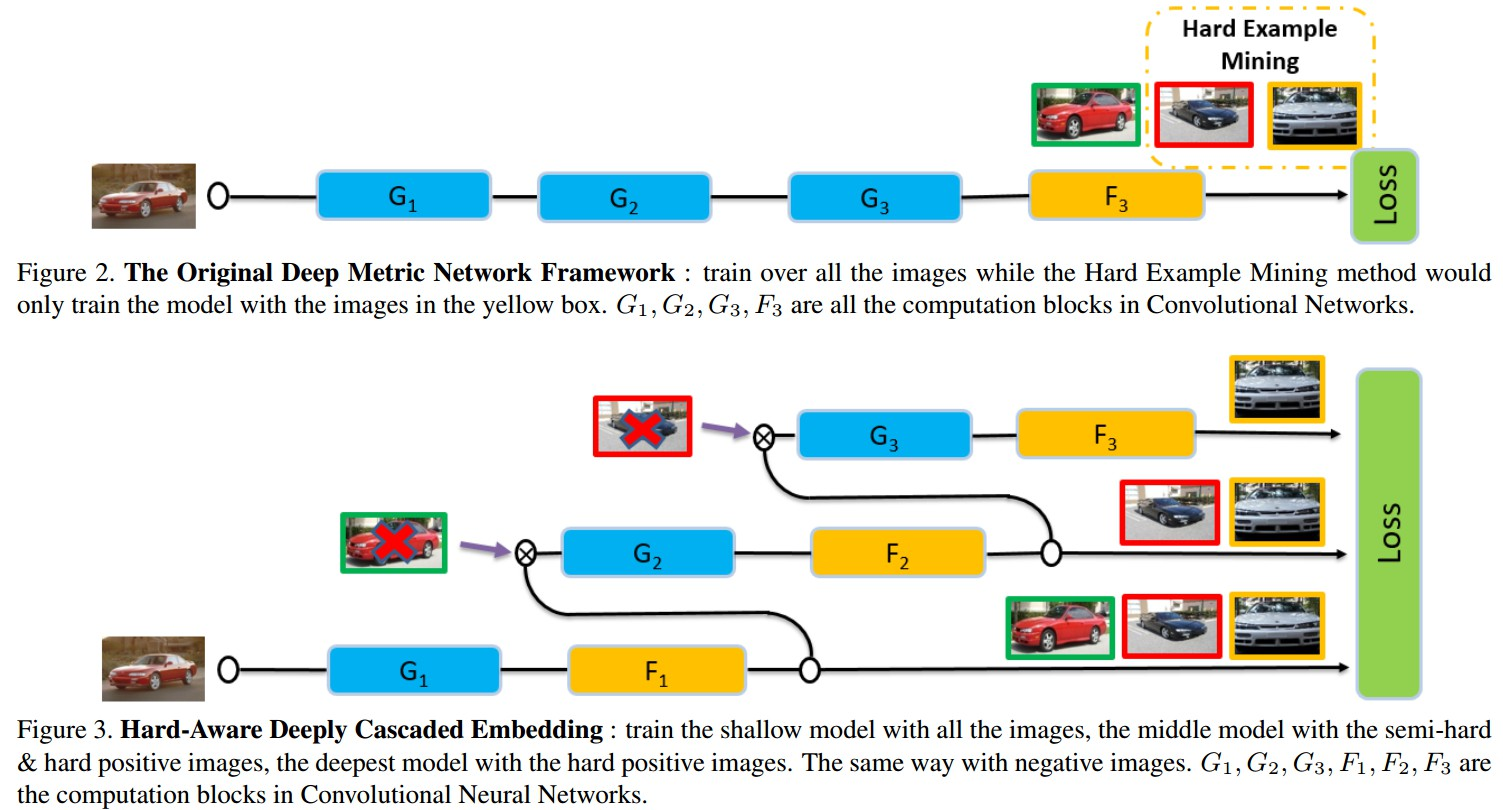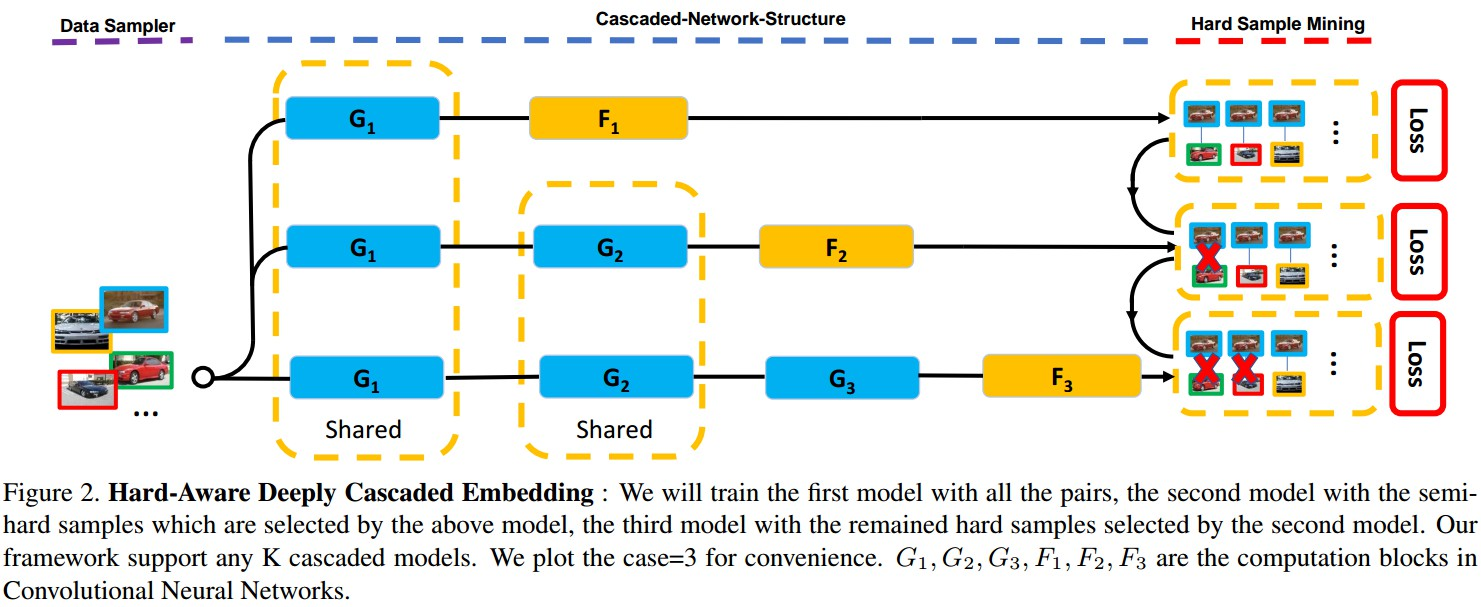Figure 2. HDC Embedding. 对所有的样本对训练模型 ${ G_1 }$，基于模型 ${ G_1 }$ 选择的 semi-hard samples 训练模型 ${ G_2 }$，基于模型 ${ G_2 }$ 选择的剩余的 hard samples 训练模型 ${ G_3 }$. ${ F_1, F_2, F_3 }$ 分别为 ${ G_1, G_2, G_3 }$ 的特征变换.

1.2 计算

${ {o_{i, 1}, o_{j, 1}} = G_1 \circ {I_i, I_j} }$

${ {f_{i,1}, f_{j,1}} = F_1 \circ {o_{i, 1}, o_{j, 1}} }$ # 对于样本对 ${ {I_i, I_j} }$ 计算得到的特征

${ {o_{i, k}, o_{j, k}} = G_k \circ {o_{i, k-1}, o_{j, k-1}} }$

${ {f_{i,k}, f_{j, k}} = F_k \circ {o_{i, k}, o_{j, k}} }$ # 网络 ${ G_k }$ 计算得到的特征

${ \mathcal{L} _k = \sum _{(i, j) \in \mathcal{P}_k } \mathcal{L} _{k}^{+} (i, j) + \sum_{(i, j) \in \mathcal{N}_k} \mathcal{L}_{k}^{-}(i, j) }$

${ \mathcal{P}_k }$，被先前模型判定为 hard examples 的所有 positive 样本对；
${ \mathcal{N}_k }$，被先前模型判定为 hard examples 的所有 negative 样本对；

HDC 的最终 Loss 函数：

${ \mathcal{L} = \sum_{k=1} ^{K} \lambda_k \mathcal{L}_k }$

${ \lambda _k }$ - 权重

Loss 关于模型 ${ G_k }$ 的梯度计算：

​ ${ \frac{\partial {\mathcal{L}} }{\partial{G_k} } = \sum_{l=k}^K \lambda_l \frac{\partial{\mathcal{L}_l}}{\partial{G_k}} }$ # ${ G_k }$ 的梯度计算需要对所有 ${ G_k }$ 相关的模型

Loss 关于变换 ${ F_k }$ 的梯度计算：

​ ${ \frac{\partial {\mathcal{L}} }{\partial{F_k} } =\lambda_k \frac{\partial{\mathcal{L}_l}}{\partial{F_k}} }$ # ${ F_k }$ 的梯度计算只需对模型 k，因为只对模型 k 进行了特征变换.

loss 函数 —— contrastive loss

Contrastive Loss 是使 positive 样本对和距离小于边缘参数的 negative 样本对间的距离尽可能大.

${ \mathcal{L}^{+}(i, j) = \mathcal{D}(f_i^{+}, f_j^{+}) }$

​${ \mathcal{L}^{-}(i, j) = max({0, \mathcal{M}- \mathcal{D}(f_i^{-}, f_j^{-})} }$

${ \mathcal{D} (f_i, f_j) }$ - 两个 L2-normalized 特征向量 ${ f_i }$ 和 ${ f_j }$ 间的 Euclidean 距离.
${ \mathcal{M} }$ - 边缘参数(margin)

${ \mathcal{L}_k = \sum_{(i,j) \in \mathcal{P}_k} \mathcal{D}(f_{i, k}^{+}, f_{j, k}^{+}) + \sum_{(i, j)\in \mathcal{N}_k)} max \lbrace 0, \mathcal{M}- \mathcal{D} (f_{i, k}^{-}, f_{j, k}^{-}) } \rbrace$

1.3 Hard Example 的选择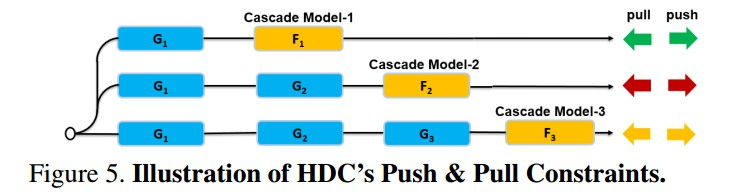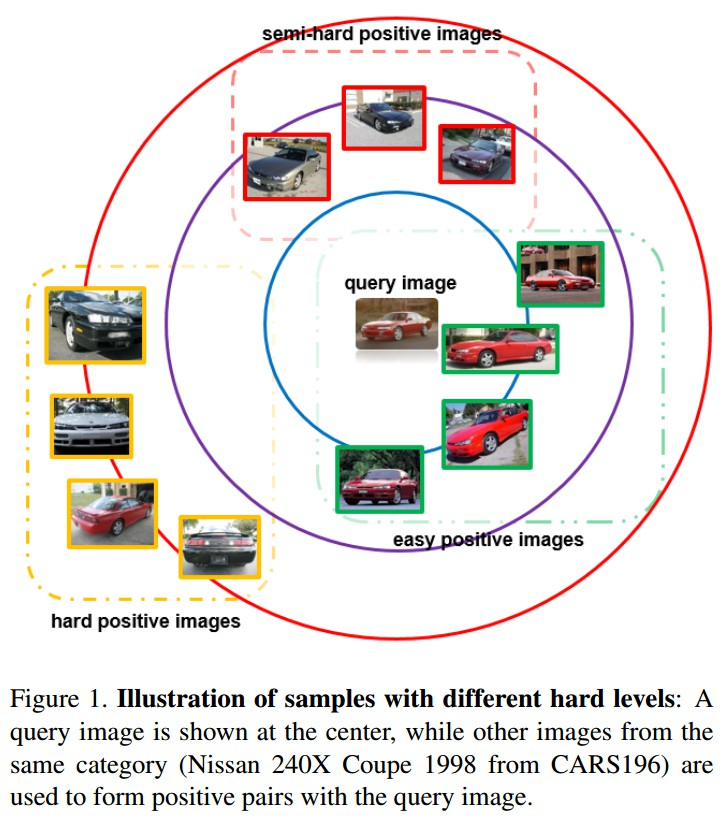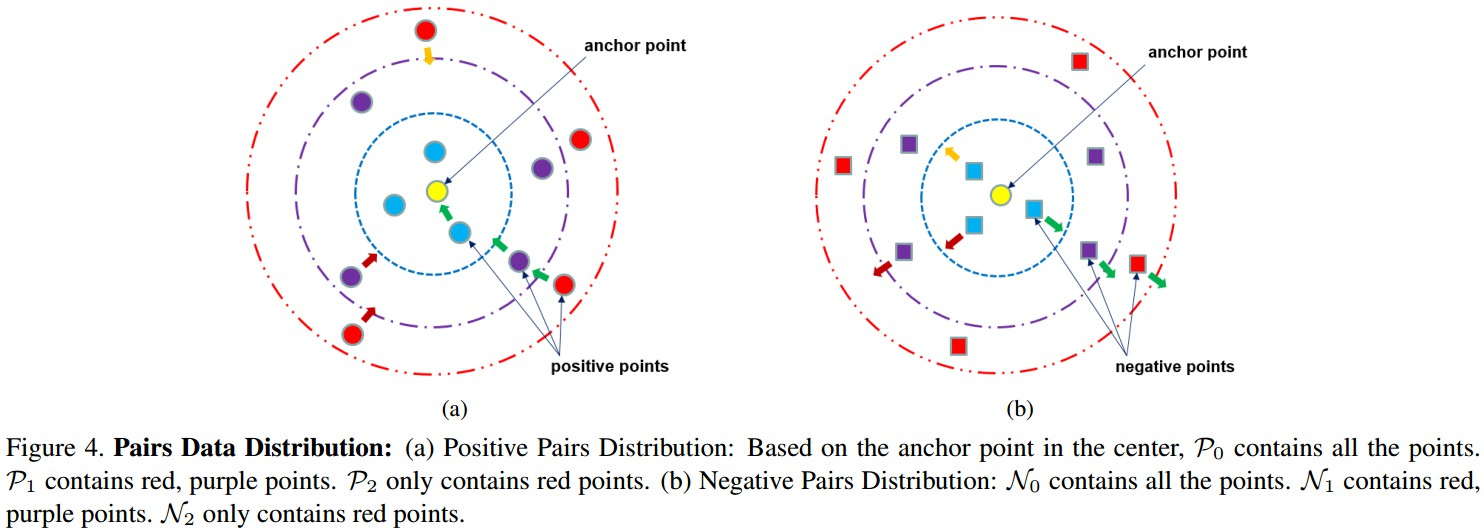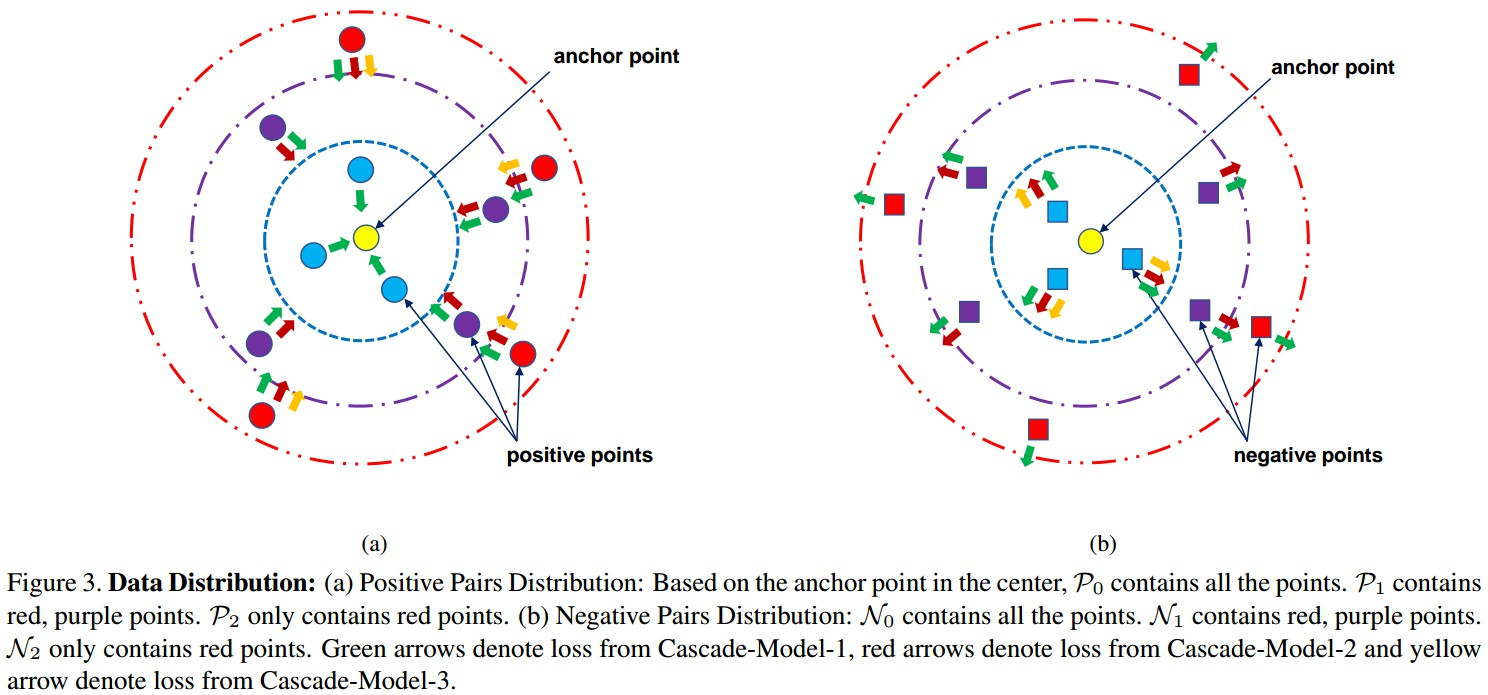Figure 3. 数据分布.

(a) Positive 样本对分布：基于中心的 anchor 点，${ \mathcal{P}_0 }$ 包含所有的点，${ \mathcal{P}_1 }$ 包含红色、紫色的点，${ \mathcal{P}_2 }$ 只包含红色的点.

(b) Negative 样本对分布： ${ \mathcal{N}_0 }$ 包含所有的点，${ \mathcal{N} _1 }$ 包含红色、紫色的点；${ \mathcal{N}_2 }$ 只包含红色的点.

Cascade-Model-1 对 ${ \mathcal{P}_0 }$ 和 ${ \mathcal{N}_0 }$ 的所有样本对进行 forward，并尝试将所有的 positive 点 push 靠近到 anchor 点，同时将所有的 negative 点 push 远离 anchor 点； 根据 loss 值选择 hard samples，形成 ${ \mathcal{P}_1 }$ 和 ${ \mathcal{N}_1 }$(即，第2个和第3个虚线圆内的点). 类似地， Cascade-Model-2 得到 ${ \mathcal{P}_2 }$ 和 ${ \mathcal{N}_2 }$(即，第3个虚线圆内的点).

1.4 HDC 实现细节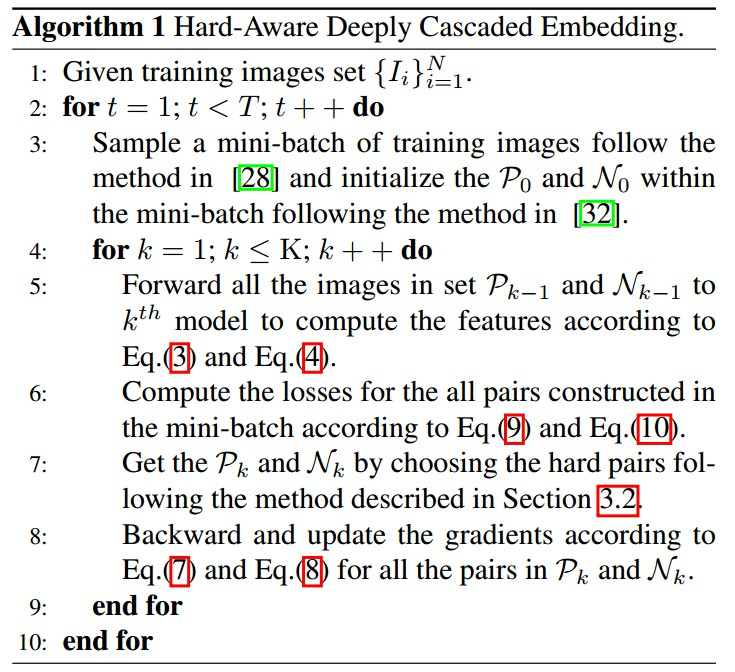2. Experiments

HDC - image-retrieval tasks.

• CARS196 dataset
196 类 cars，16185 张图片，前 98 类(8054张图片)作训练，其余 98 类(8131张图片) 作测试.
• CUB-200-2011 dataset
200 类 birds，11788 张图片，前 100 类(5864 张图片)作训练，其余的(5924 张图片) 作测试.
• Stanford Online Products dataset
22634 类 products，120053 张图片，11318 类(59551 张图片) 作训练，其余的 11316 类(共 60502 张图片)作测试.
• In-shop Clothes Retrieval dataset
DeepFashion，11735 类 clothes，54642 张图片，从中筛选 7982 类(52712 张图片) 作训练和测试. 3997 类(25882 张图片)作训练，3985类(28760 张图片)作测试.
测试集分为 query set 和 database set. query set 共 3985 类(14218 张图片)；database set 共 3985 类(12612 张图片).
• VehicleID dataset
26267 类 vehicles，221763 张图片，13134 类(110178 张图片)作训练，13133 类(111585 张图片)作测试.

2.2 Results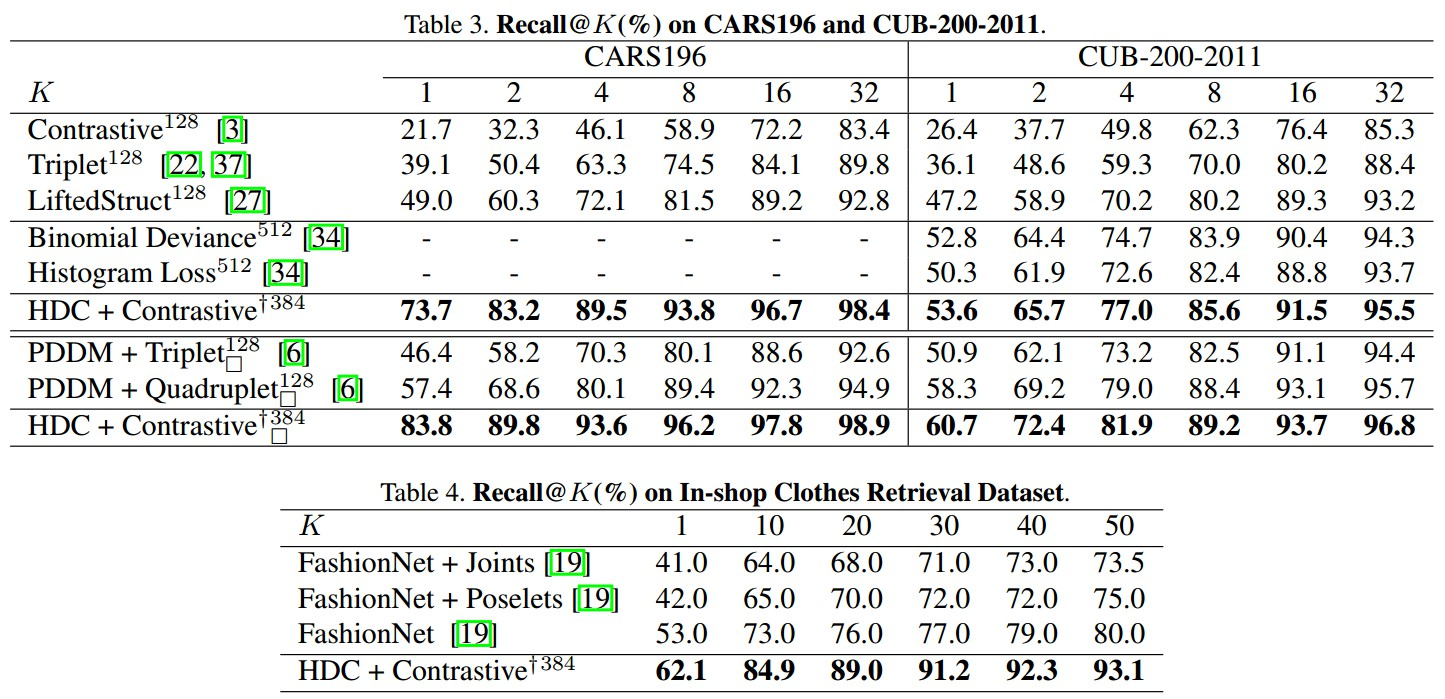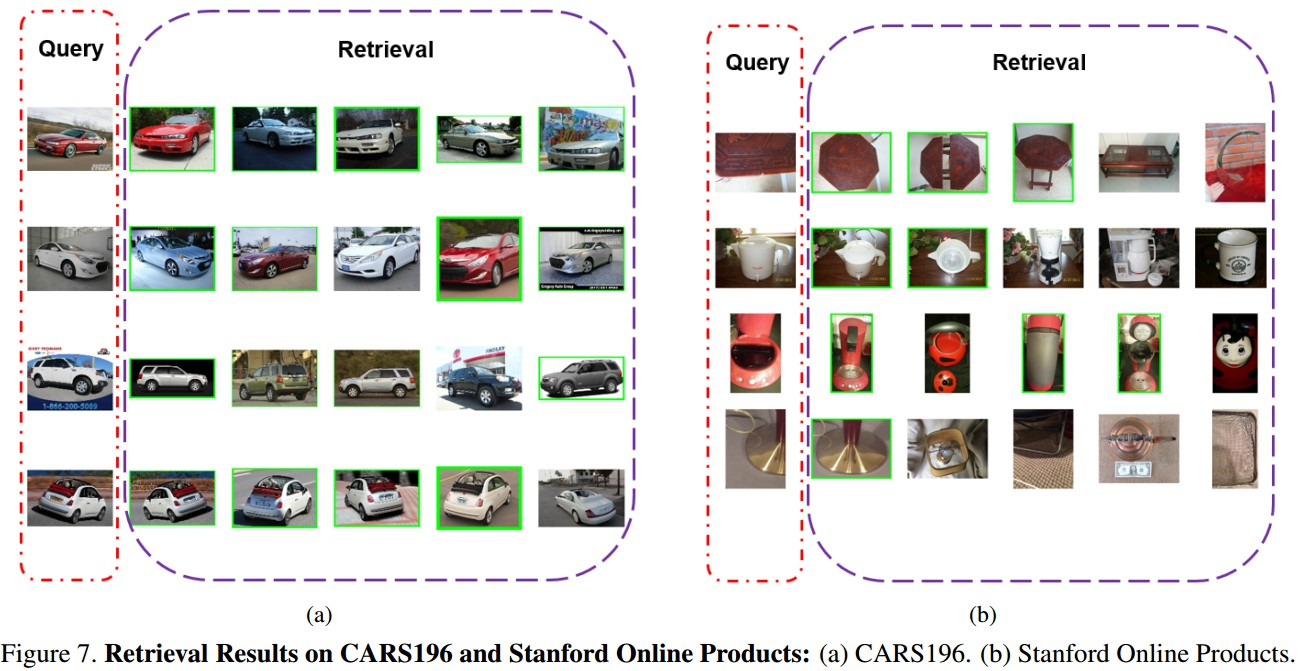Last modification：October 10th, 2018 at 04:24 pm